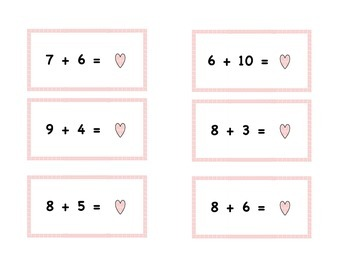# Valentine's Fact SortSubject
Resource Type
Format
PDF (1 MB|7 pages)
Standards

### Description

Valentine's Day-themed fact sort. Practice basic facts by sorting them into three categories: greater than 14, less than 14, and equal to 14.

Have you seen my other February products?

Valentine's Primary Math Packet

Valentine's Day Following Directions Lesson - freebie

Thanks for visiting my store!

Be sure to follow for information on freebies, sales, and new products.

I also blog at: Not very fancy

Deb

Total Pages
7 pages
N/A
Teaching Duration
N/A
Report this Resource to TpT
Reported resources will be reviewed by our team. Report this resource to let us know if this resource violates TpT’s content guidelines.

### Standards

to see state-specific standards (only available in the US).
Add and subtract within 20, demonstrating fluency for addition and subtraction within 10. Use strategies such as counting on; making ten (e.g., 8 + 6 = 8 + 2 + 4 = 10 + 4 = 14); decomposing a number leading to a ten (e.g., 13 - 4 = 13 - 3 - 1 = 10 - 1 = 9); using the relationship between addition and subtraction (e.g., knowing that 8 + 4 = 12, one knows 12 - 8 = 4); and creating equivalent but easier or known sums (e.g., adding 6 + 7 by creating the known equivalent 6 + 6 + 1 = 12 + 1 = 13).
Compare two two-digit numbers based on meanings of the tens and ones digits, recording the results of comparisons with the symbols >, =, and <.